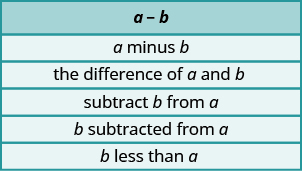## Applications With Subtracting Integers

### Learning Outcomes

• Translate statements from words into algebraic expressions involving subtraction
• Solve application problems involving subtraction

When we first introduced the operation symbols, we saw that the expression $a-b$ may be read in several ways as shown below.Be careful to get $a$ and $b$ in the right order!

### example

Translate and then simplify:

1. The difference of $13$ and $-21$
2. Subtract $24$ from $-19$

Solution
1. A difference means subtraction. Subtract the numbers in the order they are given.

 $\text{the }\color{red}{\text{difference}}\text{ of }13\text{ and }-21$ Translate. $13-(-21)$ Simplify. $34$

2. Subtract means to take $24$ away from $-19$.

 $\color{red}{\text{subtract }} 24\color{red}{\text{ from }}-19$ Translate. $-19-24$ Simplify. $-43$

### try it

Watch the following video to see more examples of how to translate an expression in words that represents subtraction into a mathematical expression.

### Subtract Integers in Applications

It’s hard to find something if we don’t know what we’re looking for or what to call it. So when we solve an application problem, we first need to determine what we are asked to find. Then we can write a phrase that gives the information to find it. We’ll translate the phrase into an expression and then simplify the expression to get the answer. Finally, we summarize the answer in a sentence to make sure it makes sense.

### Solve Application Problems

1. Identify what you are asked to find.
2. Write a phrase that gives the information to find it.
3. Translate the phrase to an expression.
4. Simplify the expression.
5. Answer the question with a complete sentence.

### example

The temperature in Urbana, Illinois one morning was $11$ degrees Fahrenheit. By mid-afternoon, the temperature had dropped to $-9$ degrees Fahrenheit. What was the difference between the morning and afternoon temperatures?

### try it

Geography provides another application of negative numbers with the elevations of places below sea level.

### example

Dinesh hiked from Mt. Whitney, the highest point in California, to Death Valley, the lowest point. The elevation of Mt. Whitney is $14,497$ feet above sea level and the elevation of Death Valley is $282$ feet below sea level. What is the difference in elevation between Mt. Whitney and Death Valley?

### try it

Managing your money can involve both positive and negative numbers. You might have overdraft protection on your checking account. This means the bank lets you write checks for more money than you have in your account (as long as they know they can get it back from you!)

### example

Leslie has $25$ in her checking account and she writes a check for $8$

1. What is the balance after she writes the check?
2. She writes a second check for $20$ What is the new balance after this check?
3. Leslie’s friend told her that she had lost a check for $10$ that Leslie had given her with her birthday card and would like her to write another one. What is the balance in Leslie’s checking account now?